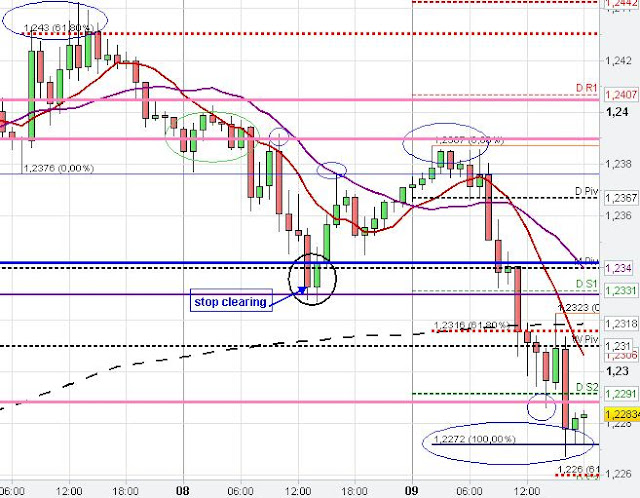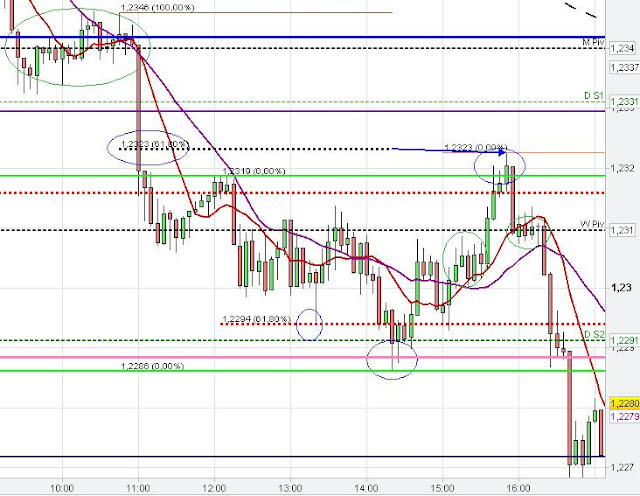## Important Fibonacci levels

61.80 Fib Retracement | 61.80 % and 100 % Fib Extension levels

#### Understanding Fibonacci Analysis

The charts below show the importance of the Fibonacci Analysis, which consists of Fibonacci Retracement levels as well as Fibonacci Extension levels.

Fibonacci Retracement levels highlight some important price levels during the retracement of a recent swing, which could lead to some price reaction. The 61.80 % Fibonacci retracement is the most important price retracement level (IMO).

The Fibonacci Extension level is the complete price range of a recent swing moved to the highest retracement level of this recent swing to target important price levels during the next impulsive leg, Hence the Fibonacci Extension levels can be drown when the retracement of the recent impulsive swing has terminated. The deepest price retracement level is the price point where the complete price range of the recent impulsive swing gets plotted on to show hidden Support/ Resistance levels. Important Fibonacci Extension levels are the 61.80 % Extension and the 100 % Extension. A minor Fibonacci Extension level is the 161 % level.

The Fibonacci Retracement gets employed first to find important price retracement levels when a recent swing is getting retraced whereby the Fibonacci Extension gets employed when the retracement terminates and a new impulsive leg starts to find important hidden support/ resistance.

On the 1 hour chart below we see that the 100 % Fibonacci Extension from the first leg down plotted on the beginning of the next leg down marks the price zone, where the EUR/USD found hidden Support after market breached the daily S2 Pivot.1 hour Fibonacci Extension

On the 5 min chart below the 61.80 % Fibonacci Retracement provided resistance to the price retracement of the larger leg down (blue arrow). Further, the leg down consists of three minor swings/ waves down,  whereby momentum was fading away with every new swing. The retracement of the first leg down started at the daily support level (pink line) after market failed to break the daily S2 Pivot.5 min Fibonacci Retracement

##### 0 Comments for "Fibonacci Retracements and Fibonacci Extensions"